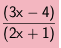Home > AC > Chapter 11 > Lesson 11.1.5 > Problem11-56

11-56.

Simplify the rational expression below. What values can x not be? Homework Help ✎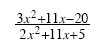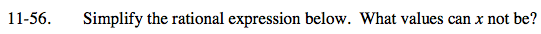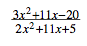The denominator cannot be equal to zero.What value(s) of x would make the denominator zero?

(2x + 1)(x + 5) = 0

2x2 + 11x + 5 = 0

x ≠ −0.5 or −5

Factor both the numerator and the denominator.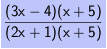Look for 1.